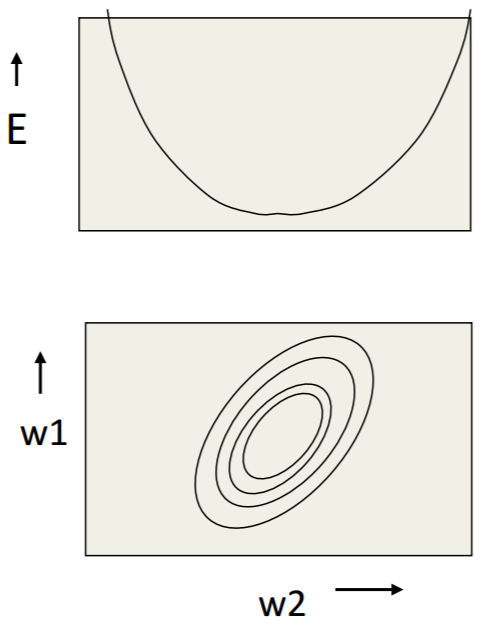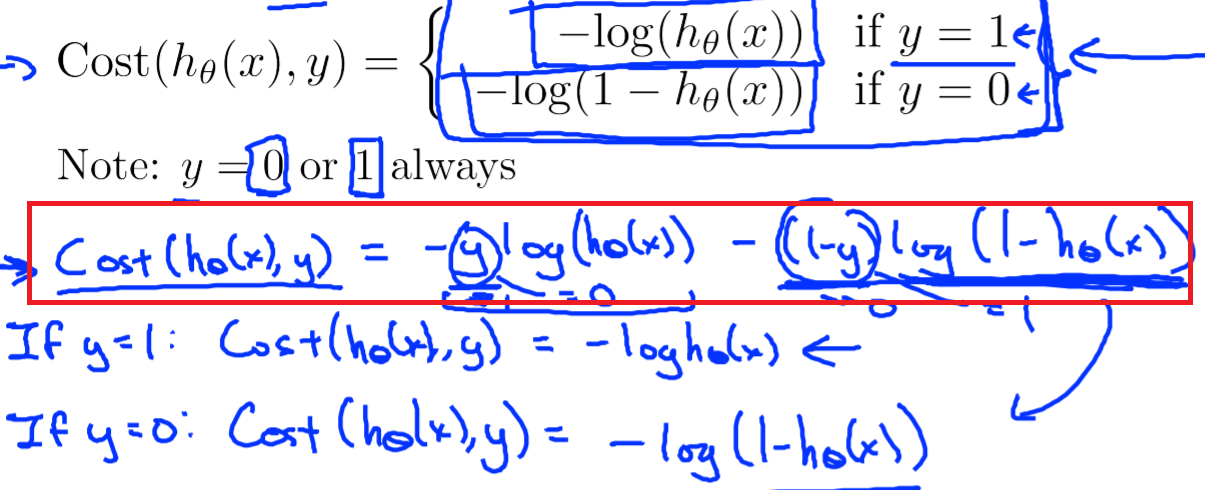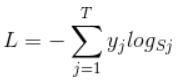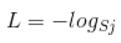# 28. Loss of the Neural Network¶

## 28.1. Error Surface&等高线¶

• 在Hilton和Andrew的课中，多次提及这个概念。
• 在图中，可以看出weights的运行轨迹。
• 下图就是一个linear neuron的error surface的垂直截面图和水平截面图，水平轴是each weight，垂直轴是error。• 梯度下降法的作用就是不断调整参数，使得模型的误差由“碗沿”降到“碗底”，参数由椭圆外部移动到椭圆的中心附近。

## 28.4. 回归场景的loss¶

IN regression problem, we employ the Euclidean(欧几里德) loss for each sample.

（from Andrew Ng Week6）### 28.4.1. 在tf中的实现¶

 ```1 2 3 4``` ```#shape of bbox_pred is [batch,4] square_error = tf.square(bbox_pred-bbox_target) #@shape(square_error)=[batch_size] square_error = tf.reduce_sum(square_error,axis=1) ```

## 28.5. 二分类场景的loss¶## 28.6. 多分类场景的loss¶

### 28.6.1. loss when trainning¶TF提供了两种方法：

### 28.6.2. loss when testing¶

《tf实战》p91 tf.nn.in_top_k()

## 28.7. OHEM(Online Hard Sample Mining)¶

MTCNN给出了OHEM的解释： In particular, in each mini-batch, we sort the loss computed in the forward propagation phase from all samples and select the top 70% of them as hard samples. Then we only compute the gradient from the hard samples in the backward propagation phase. That means we ignore the easy samples that are less helpful to strengthen the detector while training.

 ```1 2 3 4 5 6 7``` ```#这段代码改编自《tf实战》p90 #mini-batch learning method #shape of cross_entropy is [batch_size] cross_entropy = tf.nn.sparse_softmax_cross_entropy_with_logits() #OHEM其实就是多加了如下的这一步计算而已 cross_entropy = tf.nn.top_k(cross_entropy, k) cross_entropy_mean = tf.reduce_mean(cross_entropy) ```